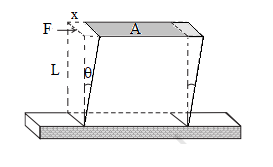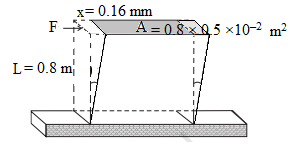# Shear Modulus Formula

## Shear Modulus Formula

Shear modulus tells how effectively a body will resist the forces applied to change its shape. When a shear force is applied on a body which results in its lateral deformation, the elastic coefficient is called the shear modulus of elasticity. It measures the rigidity of a body. Conceptually, it is the ratio of shear stress to shear strain in a body.
Let, on application of a force F tangentially on the top surface of a box fixed at the bottom, the top surface get displaced by x and a plane perpendicular to the force get turned by an angle $\theta$as shown.Then, the shear stress: $\sigma = \frac{F}{A}$
Shear strain: $\theta = \frac{x}{L}$ (As $\theta$ is very very small, $\tan \theta = \theta$)
L is the perpendicular distance (on a plane perpendicular to the force) to the layer that gets displaced by an extent x, from the fixed layer.
Then, shear modulus: $G = \frac{{shear\,\,\,stress}}{{shear\,\,\,strain}} = \frac{{F/A}}{{x/L}} = \frac{{FL}}{{Ax}}$
Unit of shear modulus is Nm–2 or pascals (Pa).
Example 1
A thin square plate of dimensions 80 cm × 80 cm × 0.5 cm is fixed vertical on one of its smaller surfaces. It is subjected to a shearing force of 2.8 ×104 N at the top. The top face of the cube is displaced through 0.16 mm with respect to the bottom surface. Find the shear modulus of elasticity of the material of the plate.
Solution:
The situation is shown in the figure.F = 2.8 ×104 N, x = 0.16 mm = 0.16 ×10–3 m, L = 0.8 m, A = 0.8 × 0.5 ×10–2 m2, G = ?
$G = \frac{{FL}}{{Ax}} = \frac{{2.8 \times {{10}^4} \times 0.8}}{{0.8 \times 0.5 \times {{10}^{--2}} \times 0.16 \times {{10}^{--3}}}} = 3.5 \times {10^{10}}Pa$

Practice question
On the application of a shear force of 8 kN on a cube of edge 4 cm and made of material of shear modulus 2 ×109 Pa, the upper face of a cube gets displaced by:
(a) 0.1 mm (b) 0.1 cm (c) 0.4 mm (d) 0.2 cm
Ans (a)